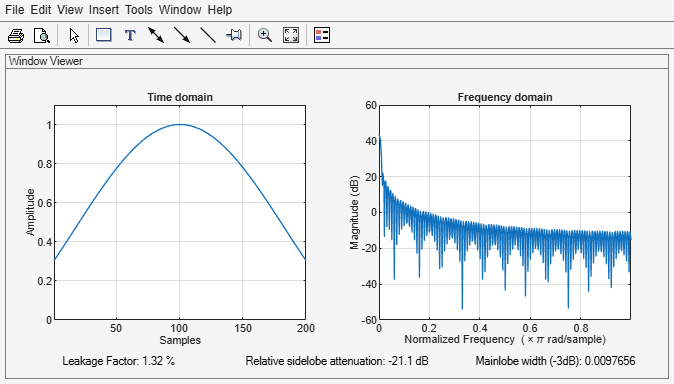Documentation

### This is machine translation

Mouseover text to see original. Click the button below to return to the English version of the page.

Note: This page has been translated by MathWorks. Click here to see
To view all translated materials including this page, select Country from the country navigator on the bottom of this page.

# kaiser

## Syntax

``w = kaiser(L,beta)``

## Description

example

````w = kaiser(L,beta)` returns an `L`-point Kaiser window with shape factor `beta`.```

## Examples

collapse all

Create a 200-point Kaiser window with a beta of 2.5. Display the result using `wvtool`.

```w = kaiser(200,2.5); wvtool(w)```## Input Arguments

collapse all

Window length, specified as a positive integer.

Data Types: `single` | `double`

Shape factor, specified as a positive real scalar. The parameter `beta` affects the sidelobe attenuation of the Fourier transform of the window.

Data Types: `single` | `double`

## Output Arguments

collapse all

Kaiser window, returned as a column vector.

## Algorithms

The coefficients of a Kaiser window are computed from the following equation:

`$w\left(n\right)=\frac{{I}_{0}\left(\beta \sqrt{1-{\left(\frac{n-N/2}{N/2}\right)}^{2}}\right)}{{I}_{0}\left(\beta \right)},\text{ }0\le n\le N,$`

where I0 is the zeroth-order modified Bessel function of the first kind. The length L = N + 1. `kaiser(L,beta)` is equivalent to

`besseli(0,beta*sqrt(1-(((0:L-1)-(L-1)/2)/((L-1)/2)).^2))/besseli(0,beta)`

To obtain a Kaiser window that represents an FIR filter with sidelobe attenuation of α dB, use the following β.

`$\beta =\left\{\begin{array}{ll}0.1102\left(\alpha -8.7\right),\hfill & \alpha >50\hfill \\ 0.5842{\left(\alpha -21\right)}^{0.4}+0.07886\left(\alpha -21\right),\hfill & 50\ge \alpha \ge 21\hfill \\ 0,\hfill & \alpha <21\hfill \end{array}$`

Increasing β widens the mainlobe and decreases the amplitude of the sidelobes (i.e., increases the attenuation).

 Kaiser, James F. “Nonrecursive Digital Filter Design Using the I0-Sinh Window Function.” Proceedings of the 1974 IEEE® International Symposium on Circuits and Systems. April, 1974, pp. 20–23.

 Digital Signal Processing Committee of the IEEE Acoustics, Speech, and Signal Processing Society, eds. Selected Papers in Digital Signal Processing. Vol. II. New York: IEEE Press, 1976.

 Oppenheim, Alan V., Ronald W. Schafer, and John R. Buck. Discrete-Time Signal Processing. Upper Saddle River, NJ: Prentice Hall, 1999, p. 474.